# A Sinous Violin¶

The aim of this short notebook is to show how to use NumPy and SciPy to play with spectral audio signal analysis (and synthesis).

Lots of prior knowledge is assumed, and here no signal theory (nor its mathematical details) will be discussed. The reader interested a more formal discussion is invited to read, for example: "Spectral Audio Signal Processing" by Julius O. Smith III that is a precise and deep, yet manageable, introduction to the topic.

For the reader less inclined in formal details (heaven forbid that the others will read the following sentence) suffices it to say that any (periodic) signal can be obtained as a superposition of sine waves (with suitable frequencies and amplitures).

The roadmap of what we'll be doing is:

• take a real signal (a violin and a flute sample),
• perform a spectral analysis,
• determine some of the frequencies having the strongest amplitudes in such spectrum,
• "reconstruct" a signal using just a few sine waves,
• play the orignal, and reconstructed signal.

As you'll see, beside what theory guarantees, this actually works and very few waves are enough to approximate the timbre of a musical instrument.

The source notebook is available on GitHub (under GPL v3), feel free to use issues to point out errors, or to fork it to suggest edits.

A special thanks to the friend and colleague Federico Pedersini for tolerating my endless discussion and my musings.

## The usual notebook setup¶

Beside the already mentioned NumPy and SciPy, we'll use librosa to read the WAV files containing the samples, and matplotlib because a picture is worth a thousand words; to play the samples we'll use the standard Audio display class of IPython.

In :
%matplotlib inline

from IPython.display import Audio
import librosa
import matplotlib.pyplot as plt
import numpy as np
import scipy as sp

plt.rcParams['figure.figsize'] = 8, 4
plt.style.use('ggplot')


## Let's begin¶

We'll fix the sampling rate once and for all to 8000Hz, that is sufficient for our audio purposes, yet low enough to reduce the number of samples involved in the following computations.

In :
RATE = 8000


We define a couple of helper functions, to load the samples in a WAV file and to generate a sine wave of given frequency and duration (given the sampling RATE defined above).

In :
def load_signal_wav(name):
signal, _ = librosa.load(name + '.wav', sr = RATE, mono = True)
return signal

In :
def sine_wave(freq, duration):
return np.sin(np.arange(0, duration, 1 / RATE) * freq * 2 * np.pi)


Let's check we've done a good job by playing a couple of seconds of a "pure A", that is a sine wave at 440hz

In :
samples_sine = sine_wave(440, 2)
Audio(samples_sine, rate = RATE)

Out:

Similarly, let's load our violin sample and play it

In :
samples_original = load_signal_wav('violin')
Audio(samples_original, rate = RATE)

Out:

## Some analysis¶

Using the specgram function we can plot a spectrogram

In :
plt.specgram(samples_original, Fs = RATE);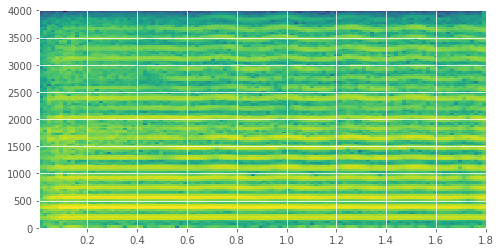even specifing just (the sampling frequency) Fs, that is the only required parameter, and without fiddling with all the others, we can already see qualitatively that there are just a few relevant frequencies (the yellow lines).

To get the precise values (and amplitudes) of such frequencies we'll need a more quantitative tool, namely the scipy.fftpack.fft function that performs a Fast Fourier Transform, and the helper function scipy.fftpack.fftfreq that locates the actual frequencies used by the FFT computation.

In :
N = samples_original.shape
spectrum = sp.fftpack.fft(samples_original)
frequencies = sp.fftpack.fftfreq(N, 1 / RATE)


Since the signal is real (that is, is made of real values), we need just the first half of the returned values; moreover (even if the theory says that the phases also matter), we are interested just in the amplitudes of the spectrum

In :
frequencies = frequencies[:N//2]
amplitudes = np.abs(spectrum[:N//2])


Plotting the result makes it evident that, in accordance with what we observed in the spectrogram, there are just a few peaks

In :
plt.plot(frequencies, amplitudes);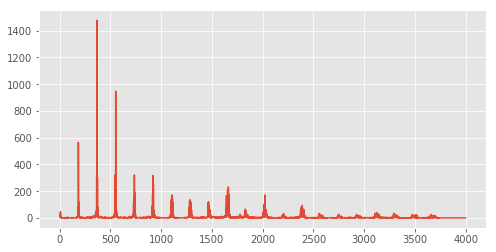### Locating the maxima¶

To find the frequencies where such peaks are located turns out to be a little tricky: to locate the peaks the scipy.signal.find_peaks_cwt needs a widths parameter specifing "the expected width of peaks of interest".

After some trial and error, one can see that 60 is a reasonable width to get close enough to the actual peaks.

In :
peak_indices = sp.signal.find_peaks_cwt(amplitudes, widths = (60,))


but plotting the peaks reveals that sometimes they are a bit off

In :
plt.plot(frequencies, amplitudes)
plt.plot(frequencies[peak_indices], amplitudes[peak_indices], 'bx');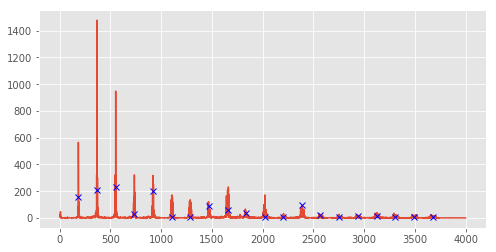let's look at 10 values around the located peaks to get the actual maxima of the amplitudes, and then use such values to locate the frequencies where they are attained

In :
amplitudes_maxima = list(map(lambda idx: np.max(amplitudes[idx - 10:idx + 10]), peak_indices))
frequencies_maxima = frequencies[np.isin(amplitudes, amplitudes_maxima)]


by plotting these values we can tell we did a good job; using a logarithmic scale we can better appreciate that also the few last values correspond to actual peaks (albeit of much smaller amplitude)

In :
plt.semilogy(frequencies, amplitudes)
plt.plot(frequencies_maxima, amplitudes_maxima, 'bx');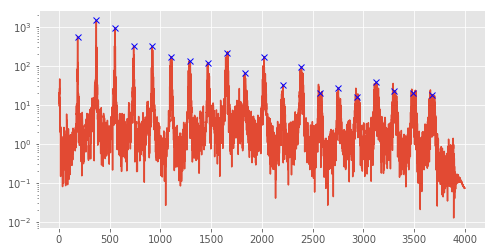We can isolate our peak finding function for further use

In :
def find_peaks(frequencies, amplitudes, width, lookaround):
peak_indices = sp.signal.find_peaks_cwt(amplitudes, widths = (width,))
amplitudes_maxima = list(map(lambda idx: np.max(amplitudes[idx - lookaround:idx + lookaround]), peak_indices))
frequencies_maxima = frequencies[np.isin(amplitudes, amplitudes_maxima)]
return frequencies_maxima, amplitudes_maxima


## Finally the synthesis¶

Now that we have both the relevant frequencies and amplitudes, we can put together the sine waves and build an approximation of the original signal

In :
def compose_sine_waves(frequencies, amplitudes, duration):
return sum(map(lambda fa: sine_wave(fa, 2) * fa, zip(frequencies, amplitudes)))

In :
samples_reconstructed = compose_sine_waves(frequencies_maxima, amplitudes_maxima, 2)


The spectrogram looks promising

In :
plt.specgram(samples_reconstructed, Fs = RATE);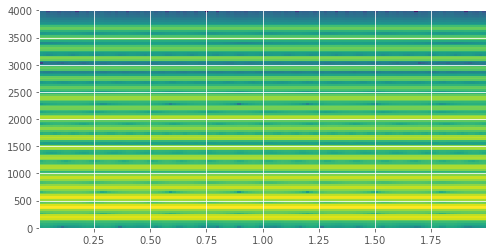but what is striking is how similar the reconstructed sound is with respect to the original one

In :
Audio(samples_reconstructed, rate = RATE)

Out:

exspecially if you compare it with just the sine wave corresponding to the maximum amplitude

In :
Audio(sine_wave(frequencies_maxima[np.argmax(amplitudes_maxima)], 2), rate = RATE)

Out:

## Not just violins¶

Of course the same game can be played with other samples, let's try a flute

In :
samples_original = load_signal_wav('flute')
Audio(samples_original, rate = RATE)

Out:

We can replicate the steps to obtain the relevant frequenceis and amplitudes, plotting the result as a quick check

In :
N = samples_original.shape
frequencies = sp.fftpack.fftfreq(N, 1 / RATE)[:N//2]
amplitudes = np.abs(sp.fftpack.fft(samples_original))[:N//2]

frequencies_maxima, amplitudes_maxima = find_peaks(frequencies, amplitudes, 100, 50)
plt.plot(frequencies, amplitudes)
plt.plot(frequencies_maxima, amplitudes_maxima, 'bx');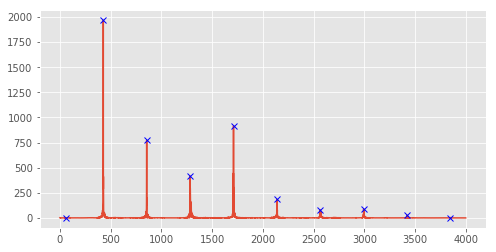and again, play the obtained sound, compared to the maximum amplitude sine wave

In :
samples_reconstructed = compose_sine_waves(frequencies_maxima, amplitudes_maxima, 2)
Audio(samples_reconstructed, rate = RATE)

Out:
In :
Audio(sine_wave(frequencies_maxima[np.argmax(amplitudes_maxima)], 2), rate = RATE)

Out:

## Wrap up¶

As promised, this notebook shows how:

• take a real signal,
• perform the Fast Fourier Transform,
• locate the frequencies corresponding to the peaks of the amplitudes in such spectrum,
• use such values to build a synthetic signal.

Even if the result is exactly what one can expect from the theory, the notebook provides a couple of quite surprising examples where a few sine waves can convey the timbre of two very different musical instruments such as a violin and a flute.